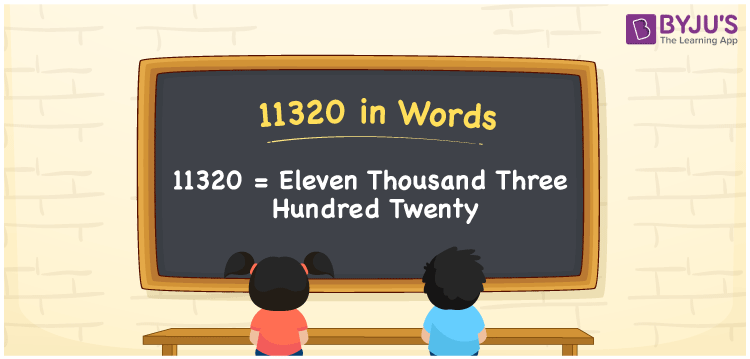# 11320 in Words

We can write 11320 in words as Eleven thousand three hundred twenty. If you bought a new pair of chairs that cost Rs. 11320, then you can say, “I bought a new pair of chairs worth Eleven thousand three hundred twenty rupees”. We can effortlessly convert the number 11320 into words with the help of a place value chart. In this article, you will learn how to write 11320 in words, along with some interesting facts about 11320.

 11320 in words Eleven thousand three hundred twenty Eleven thousand three hundred twenty in numerals 11320

## 11320 in English Words

Generally, we express numbers in words using the English alphabet. So, we spell 11320 in English words as “Eleven thousand three hundred twenty”.## How to Write 11320 in Words?

The number 11320 has five digits. Let us create a place value chart with columns to assign a place value for each of the five digits of the number 11320, as shown below.

 Ten thousands Thousands Hundreds Tens Ones 1 1 3 2 0

Here, ones = 0, tens = 2, hundreds = 3, thousands = 1, ten thousands = 1

The above numbers can be expanded as follows.

1 × Ten thousand + 1 × Thousand + 3 × Hundred + 2 × Ten + 0 × One

= 1 × 10000 + 1 × 1000 + 3 × 100 + 2 × 10 + 0 × 1

= 10000 + 1000 + 300 + 20

= Ten Thousand + One thousand + Three hundred + Twenty

= Eleven thousand + Three hundred twenty

= Eleven thousand three hundred twenty

11320 is a natural number that is the successor of 11319 and predecessor of 11321.

11320 in words – Eleven thousand three hundred twenty

Is 11320 an odd number? – No

Is 11320 an even number? – Yes

Is 11320 a prime number? – No

Is 11320 a composite number? – Yes

Is 11320 a perfect square number? – No

Is 11320 a perfect cube number? – No

## Frequently Asked Questions on 11320 in Words

Q1

### How do you write 11320 in words?

The number 11320 can be written in words as Eleven thousand three hundred twenty.
Q2

### How to write Rs. 11320 in words on a cheque?

On a cheque, an amount of Rs. 11320 can be written in words as “Eleven thousand three hundred twenty rupees only”.
Q3

### Find the difference between Eleven thousand three hundred twenty and Six thousand three hundred twenty.

Eleven thousand three hundred twenty minus Six thousand three hundred twenty = 11320 – 6320 = 5000, i.e., Five thousand.
Test your Knowledge on 11320 in Words